# RImagePalette### Extract colors from an image, then use them in plots or for fun!

The `RImagePalette` package is a pure R implementation of the median cut algorithm for extracting the dominant colors from an image. This package lets you use the colors from an image you like to create pretty plots, or to swap colors from one image to another.

Install from CRAN using:

``install.packages("RImagePalette")``

Or from github, using:

``devtools::install_github("joelcarlson/RImagePalette")``

# Viewing Palettes

It’s simple to create palettes from an image using the `image_palette()` function:

``````library(RImagePalette)

display_image(lifeAquatic)````````````#Create a palette of 9 colors
lifeAquaticPalette <- image_palette(lifeAquatic, n=9)
scales::show_col(lifeAquaticPalette)``````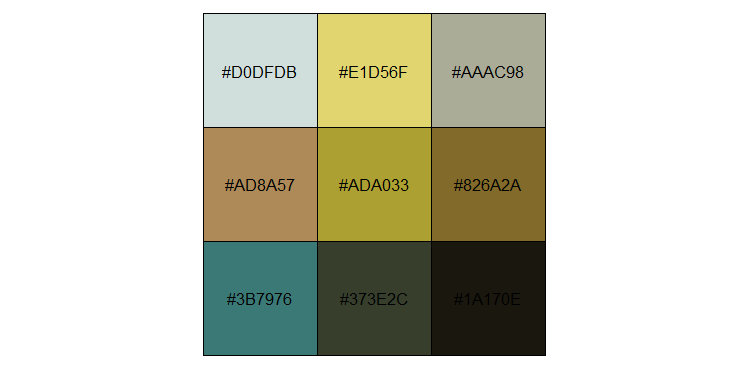Not happy with the results? We can tweak some settings until the scale is to our liking:

``````lifeAquaticPalette <- image_palette(lifeAquatic, n=9, choice=median, volume=TRUE)
scales::show_col(lifeAquaticPalette)``````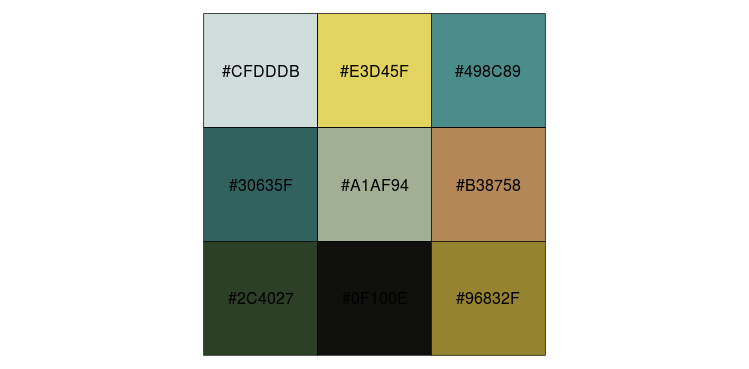If it contains colors we like, we can pick and choose, and use them as a scale:

``````library(ggplot2)
#Create plot
p <- ggplot(data = iris, aes(x=Species, y=Sepal.Width, fill=Species)) + geom_bar(stat="identity")
#Apply scale
p + theme_bw() + scale_fill_manual(values=lifeAquaticPalette[c(2,3,6)])``````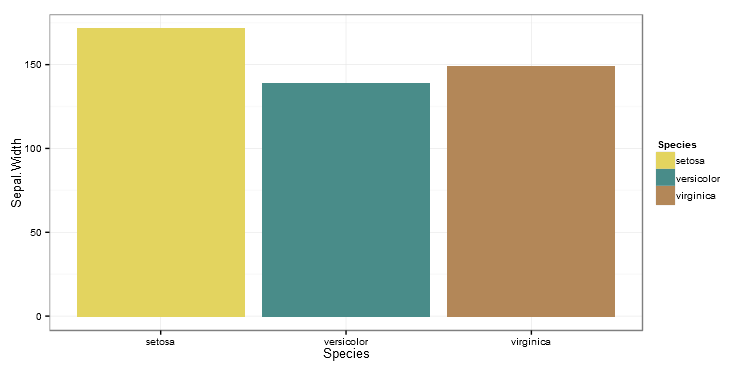# Images as Scales

`RImagePalette` can create both discrete and continuous scales from images for use with `ggplot2` using the new `scale_color_image` (or for plots requiring fills, the `scale_fill_image()`) function:

``````#Load an image
display_image(desert)``````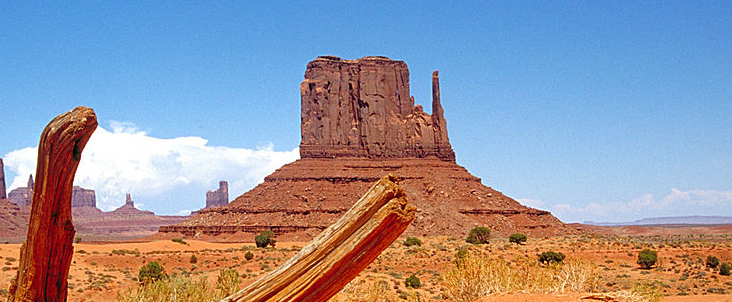### Discrete Scale

``````#Create plot
p <- ggplot(data = iris, aes(x=Sepal.Length, y=Sepal.Width, col=Species)) + geom_point(size=3)
p + theme_bw() + scale_color_image(image=desert)``````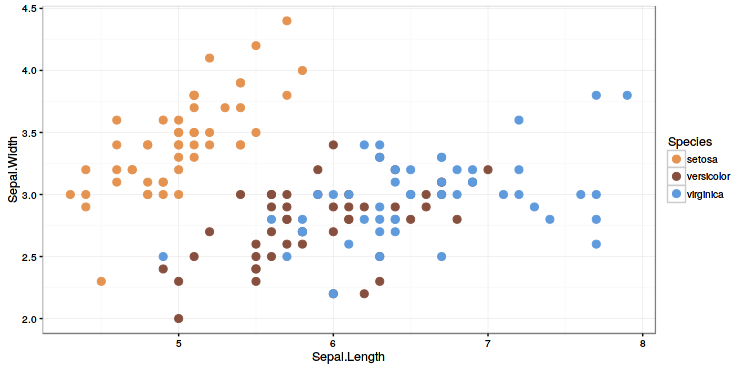### Continuous Scale

``````#Create plot
p <- ggplot(data = iris, aes(x=Sepal.Length, y=Sepal.Width, col=Sepal.Length)) + geom_point(size=3)
#Use discrete=FALSE for a continuous scale
p + theme_bw() + scale_color_image(image=desert, discrete=FALSE) ``````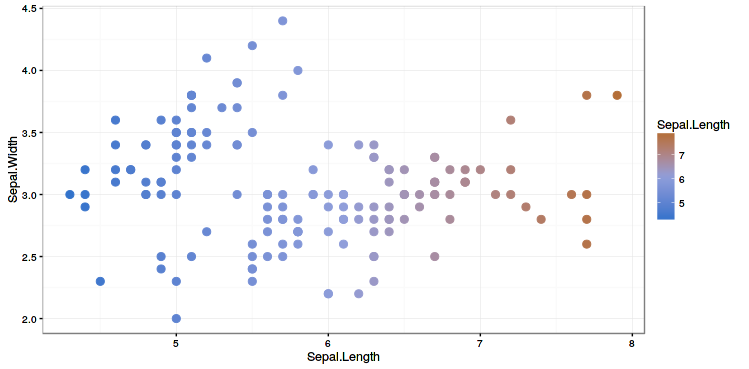# Quantizing Images

Note: This feature is experimental at the moment, and as such is non-optimized, and slow. You must install from github to access the `quantize_image` function

We can also quantize images into a discrete number of colors using the `quantize_image` function:

``````#Load the famous mandrill

#Quantize using 7 colors
quant_mandrill <- quantize_image(mandrill, n=7)``````

When displayed closely reproduces the original image: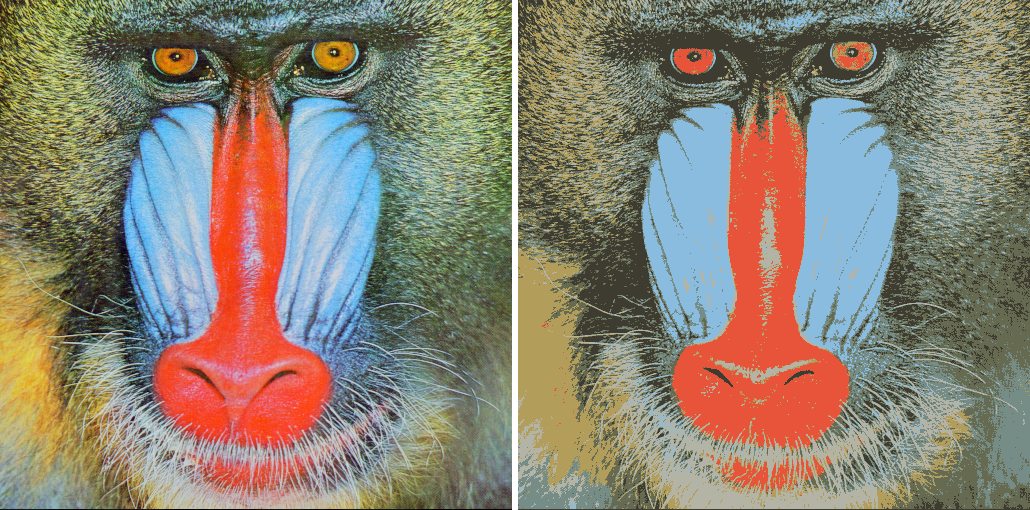Another method for doing so is to use the kmeans approach, as discussed in this blog post by Ryan Walker. Here is the comparison between kmeans (on the left) and median cut (on the right) using 4 colors: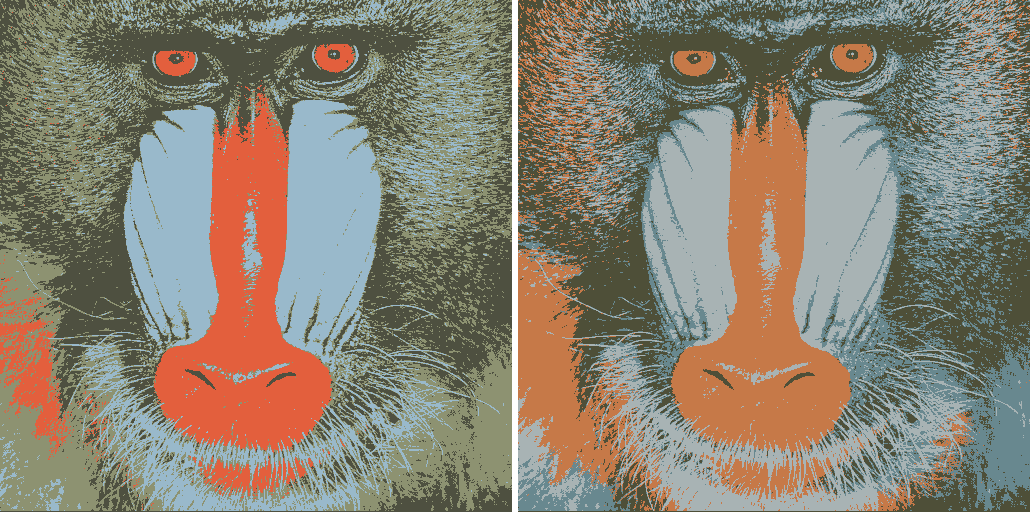# Just for fun

We can swap colors across images using the `switch_colors()` function:

``````celery <- jpeg::readJPEG("figs/CeleryLunch.jpg")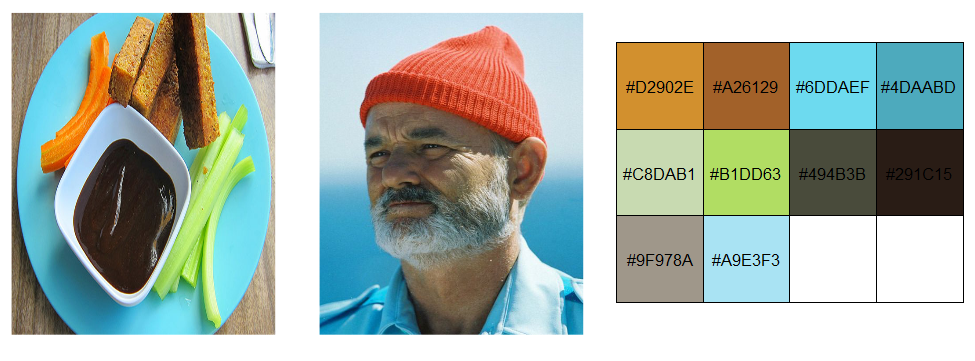``switch_colors(billMurray, celery, source_colors = 10)``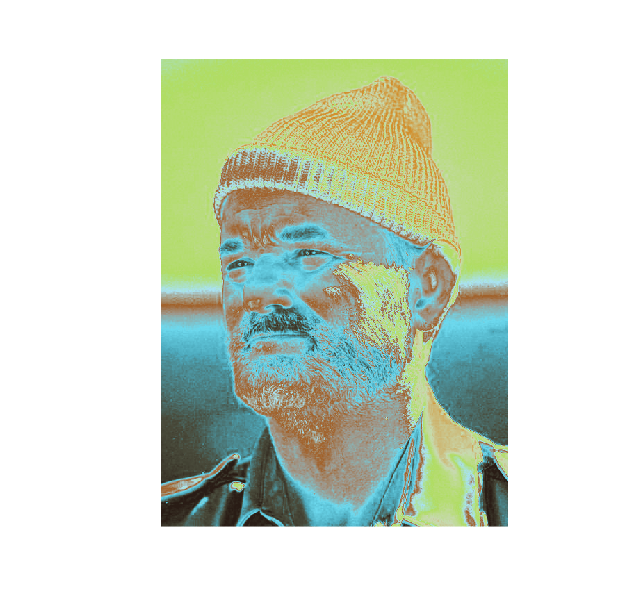### Note

There is an element of randomness in the median cut algorithm, so set your seeds carefully, and try running the algorithm a few times if you aren’t happy with the results. Other ways to alter the palette: try using `choice = median`, `volume = TRUE` or change the value of `n`.

### Special Thanks

There are a number of projects that inspired or helped this project along, and they deserve some recognition:

Thank you all for your great work!